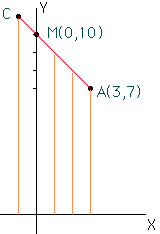Courtney Smith I am a tenth grade student I would appreciate assistance with the following problem In triangle ABC,segment MN divides sides(segment)AC and (segment)AB proportionally. If the coordinates are A(3,7),M(0,10) and N(8,22) and if AM:MC = 3:1, find the coordinates of B and C. Thank you very much Hi Courtney, Look first at the line segment AC. Horizontially, the point M is 3 units to the left of A (3 - 0 = 3). The distance from A to M is 3 times the distance from M to C so C is 1 unit to the left of M. Thus the X-coordinate of C is 0 - 1 = -1. Likewise, in the vertical direction M is 3 units above A so C is 1 unit above M and thus the Y-coordinate of C is 11. Hence C has coordinates (-1,11).Now consider CB. C is (-1,11) and N is (8,22). N is 9 units to the right of C and |NB| is 3 times |CN|, thus B is 3x9 = 27 units to the right of N. Thus the X-coordinate of B is 8 + 27 = 35. Finally N is 11 units above C and thus B is 3x11 = 33 units above N. Thus the Y-coordinate of B is 22 + 33 = 44. Cheers, Penny Go to Math Central